# Numerate

## Player 2

### 0

Drag some tiles onto the board to form an equation then click the confirm button.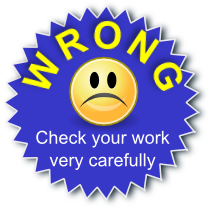Hide this message

Two players take it in turns to form equations on the board using the available tiles. The first turn of the first player should have the equation going through the centre square. For the following turns, each new equation should use at least one tile of an existing equation but any bonus (such as double symbol score) earned by the tile being reused does not apply to the new equation.

When both players agree that the equation is correct the score is calculated and recorded then the confirm button pressed.

The first player to earn 200 points is the winner

The names 'Player 1' and 'Player 2' and the scores are editable so you can keep your own score.

## Codes:

The score for each symbol can be found on the bottom right corner of the tile. The scores are increased if the tile is placed on a square with one of the following codes.

2S - Double symbol score. 3S - Triple symbol score. 4S - Quadruple symbol score.

2E - Double equation score. 3E - Triple equation score. 4E - Quadruple equation score.

## Note for Teachers

This game is designed for pupils to manage themselves. They judge whether equations formed are correct and they keep their own score. Consequently the system is unable to automatically award trophies. Teachers, logged into their Transum subscription accounts are able to award trophies to pupils who have made a particularly good effort playing this game by using the button below (which appears when you are signed in).

If you do not yet have an account and you are a teacher, tutor or parent you can apply for one by completing the form after clicking the button below.

Mandy Messenger,

Tuesday, July 10, 2018

"Why does it not calculate the scores? That stay at zero.

[Transum: Thank you for your comment Mandy. The idea is for players to calculate their own scores and type them in to the score board where the zeros are.]"

Do you have any comments? It is always useful to receive feedback and helps make this free resource even more useful for those learning Mathematics anywhere in the world. Click here to enter your comments.

#### Dump-A-Dice Race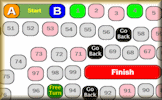An online board game for two players involving prime and square numbers and making choices.

Transum.org/go/?to=dumpdice

#### Ultimate Noughts and Crosses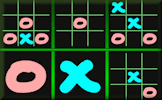A game requiring you to devise a strategy far more complex than that required for the standard game.

Transum.org/go/?to=ultimate

#### Remainder Race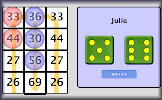A game involving chance and choice requiring an ability to calculate the remainder when a two digit number is divided by a single digit number.

Transum.org/go/?to=remainder

#### Mathopoly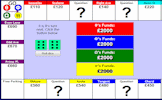A game of buying and selling property with maths questions thrown in for good measure.

Transum.org/go/?to=mathopoly

#### DiceGebra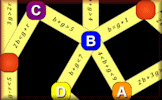An online board game for two players evaluating algebraic equations and inequalities.

Transum.org/go/?to=dgebra

#### Tran TowersAn adventure game requiring students to solve puzzles as they move through the old mansion.